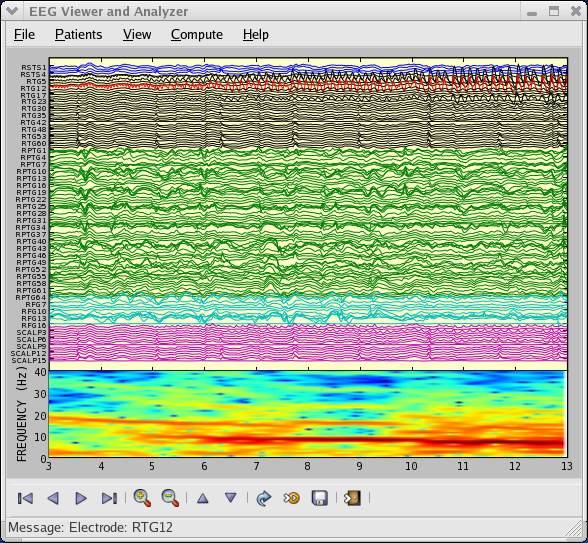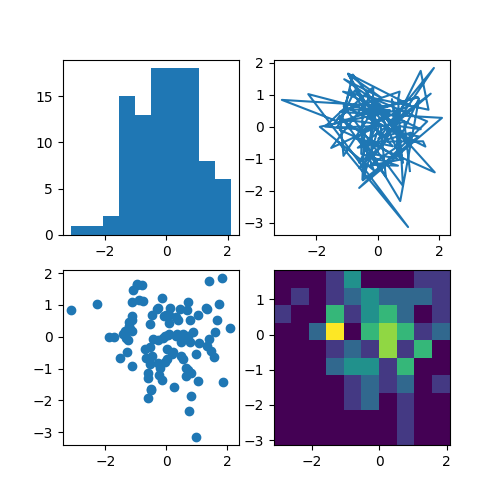You are reading an old version of the documentation (v3.1.3). For the latest version see https://matplotlib.org/stable/tutorials/introductory/sample_plots.html

# Sample plots in Matplotlib¶

Here you'll find a host of example plots with the code that generated them.

## Line Plot¶

Here's how to create a line plot with text labels using plot().

## Multiple subplots in one figure¶

Multiple axes (i.e. subplots) are created with the subplot() function:

## Images¶

Matplotlib can display images (assuming equally spaced horizontal dimensions) using the imshow() function.

## Contouring and pseudocolor¶

The pcolormesh() function can make a colored representation of a two-dimensional array, even if the horizontal dimensions are unevenly spaced. The contour() function is another way to represent the same data:

## Histograms¶

The hist() function automatically generates histograms and returns the bin counts or probabilities:

## Paths¶

You can add arbitrary paths in Matplotlib using the matplotlib.path module:

## Three-dimensional plotting¶

The mplot3d toolkit (see Getting started and 3D plotting) has support for simple 3d graphs including surface, wireframe, scatter, and bar charts.

Thanks to John Porter, Jonathon Taylor, Reinier Heeres, and Ben Root for the mplot3d toolkit. This toolkit is included with all standard Matplotlib installs.

## Streamplot¶

The streamplot() function plots the streamlines of a vector field. In addition to simply plotting the streamlines, it allows you to map the colors and/or line widths of streamlines to a separate parameter, such as the speed or local intensity of the vector field.

This feature complements the quiver() function for plotting vector fields. Thanks to Tom Flannaghan and Tony Yu for adding the streamplot function.

## Ellipses¶

In support of the Phoenix mission to Mars (which used Matplotlib to display ground tracking of spacecraft), Michael Droettboom built on work by Charlie Moad to provide an extremely accurate 8-spline approximation to elliptical arcs (see Arc), which are insensitive to zoom level.

## Bar charts¶

Use the bar() function to make bar charts, which includes customizations such as error bars:

You can also create stacked bars (bar_stacked.py), or horizontal bar charts (barh.py).

## Pie charts¶

The pie() function allows you to create pie charts. Optional features include auto-labeling the percentage of area, exploding one or more wedges from the center of the pie, and a shadow effect. Take a close look at the attached code, which generates this figure in just a few lines of code.

## Tables¶

The table() function adds a text table to an axes.

## Scatter plots¶

The scatter() function makes a scatter plot with (optional) size and color arguments. This example plots changes in Google's stock price, with marker sizes reflecting the trading volume and colors varying with time. Here, the alpha attribute is used to make semitransparent circle markers.

## GUI widgets¶

Matplotlib has basic GUI widgets that are independent of the graphical user interface you are using, allowing you to write cross GUI figures and widgets. See matplotlib.widgets and the widget examples.

## Filled curves¶

The fill() function lets you plot filled curves and polygons:

Thanks to Andrew Straw for adding this function.

## Date handling¶

You can plot timeseries data with major and minor ticks and custom tick formatters for both.

See matplotlib.ticker and matplotlib.dates for details and usage.

## Log plots¶

The semilogx(), semilogy() and loglog() functions simplify the creation of logarithmic plots.

Thanks to Andrew Straw, Darren Dale and Gregory Lielens for contributions log-scaling infrastructure.

## Polar plots¶

The polar() function generates polar plots.

## Legends¶

The legend() function automatically generates figure legends, with MATLAB-compatible legend-placement functions.

Thanks to Charles Twardy for input on the legend function.

## TeX-notation for text objects¶

Below is a sampling of the many TeX expressions now supported by Matplotlib's internal mathtext engine. The mathtext module provides TeX style mathematical expressions using FreeType and the DejaVu, BaKoMa computer modern, or STIX fonts. See the matplotlib.mathtext module for additional details.

Matplotlib's mathtext infrastructure is an independent implementation and does not require TeX or any external packages installed on your computer. See the tutorial at Writing mathematical expressions.

## Native TeX rendering¶

Although Matplotlib's internal math rendering engine is quite powerful, sometimes you need TeX. Matplotlib supports external TeX rendering of strings with the usetex option.

## EEG GUI¶

You can embed Matplotlib into pygtk, wx, Tk, or Qt applications. Here is a screenshot of an EEG viewer called pbrain.The lower axes uses specgram() to plot the spectrogram of one of the EEG channels.

For examples of how to embed Matplotlib in different toolkits, see:

## XKCD-style sketch plots¶

Just for fun, Matplotlib supports plotting in the style of xkcd.

## Subplot example¶

Many plot types can be combined in one figure to create powerful and flexible representations of data.import matplotlib.pyplot as plt
import numpy as np

np.random.seed(19680801)
data = np.random.randn(2, 100)

fig, axs = plt.subplots(2, 2, figsize=(5, 5))
axs[0, 0].hist(data)
axs[1, 0].scatter(data, data)
axs[0, 1].plot(data, data)
axs[1, 1].hist2d(data, data)

plt.show()


Keywords: matplotlib code example, codex, python plot, pyplot Gallery generated by Sphinx-Gallery